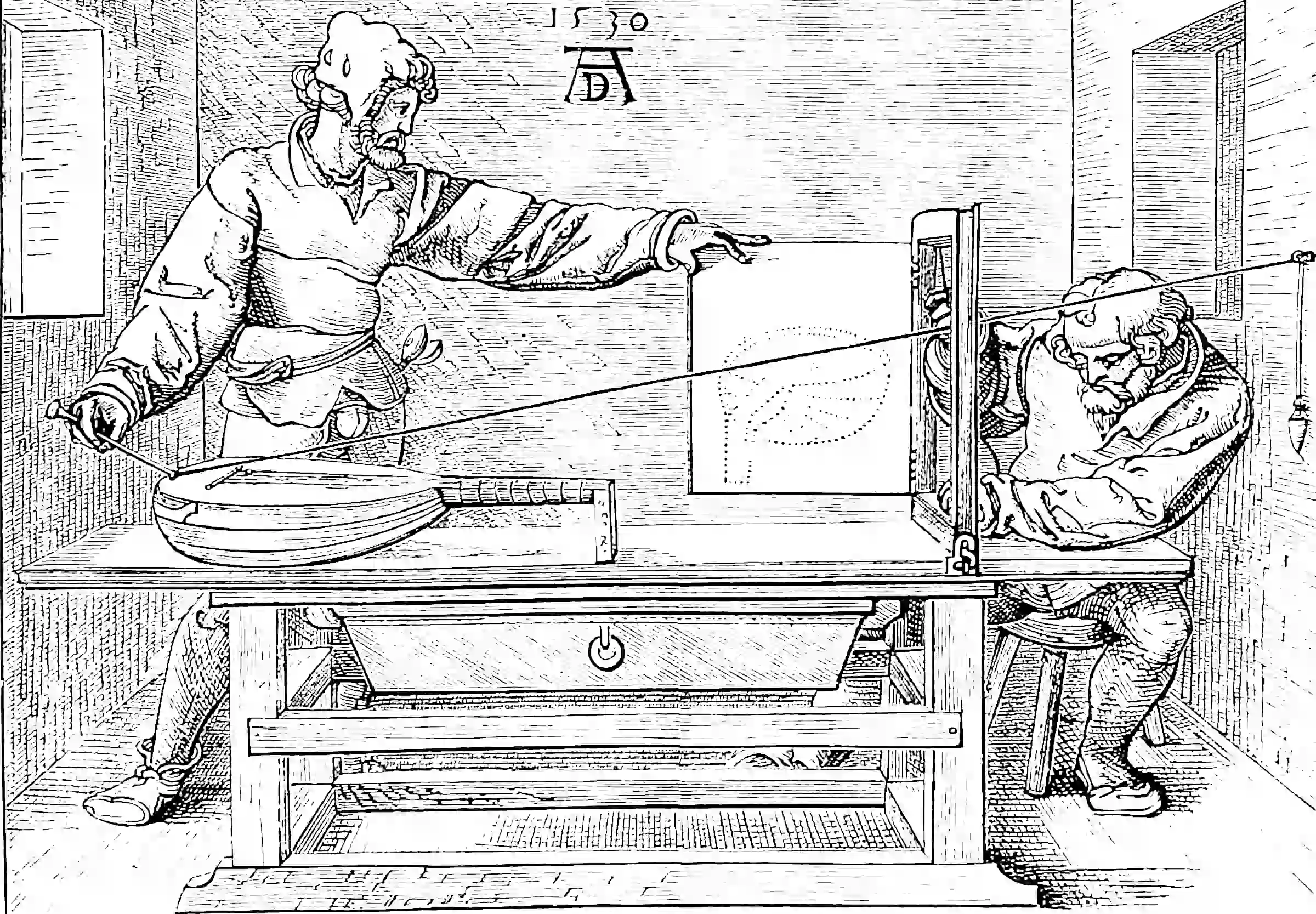# Inner product spaces

## The most highly developed theory of squaring things

$$\renewcommand{\var}{\operatorname{Var}} \renewcommand{\dd}{\mathrm{d}} \renewcommand{\pd}{\partial} \renewcommand{\bb}{\mathbb{#1}} \renewcommand{\vv}{\boldsymbol{#1}} \renewcommand{\mm}{\mathrm{#1}} \renewcommand{\mmm}{\mathrm{#1}} \renewcommand{\cc}{\mathcal{#1}} \renewcommand{\oo}{\operatorname{#1}} \renewcommand{\gvn}{\mid} \renewcommand{\II}{\mathbb{I}\{#1\}} \renewcommand{\inner}{\langle #1,#2\rangle} \renewcommand{\Inner}{\left\langle #1,#2\right\rangle} \renewcommand{\norm}{\| #1\|} \renewcommand{\Norm}{\|\langle #1\right\|} \renewcommand{\argmax}{\operatorname{arg max}} \renewcommand{\argmin}{\operatorname{arg min}} \renewcommand{\omp}{\mathop{\mathrm{OMP}}}$$

The most well-worn tool in the functional analysis kit. Let’s walk through the classic setup, as a refresher to my dusty brains. Many details will be skipped, much violence will be done to details

## Normed spaces

We work on normed spaces which are vector spaces endowed with a notion of distance.

We are considering particular types of normed spaces, which have the additional structure of inner products, and norms induced by their inner products.

But first!

We take a normed space $$\cc{F}$$, which will be a space of functions $$\cc{T}\to\cc{V}$$. When I say functions I mean ODE solutions, signals, vectors and even the trivial case of scalars, which are constant functions, but since there are functions of functions in the mix, we need to keep constant in mind what we are working with. I call $$\cc{T}$$ the index set, which we may as well take $$\mathbb{C}^N,$$ and $$\cc{V}$$ the state set, which can be $$\mathbb{C}^M$$ why not? The other case I care about often is $$\cc{T}=\mathbb{Z}$$ but… later.

### Operators

Let’s say we have two normed spaces, $$\cc{F}$$ and $$\cc{G}.$$ An operator $$\Phi$$ on $$\cc{F}$$ is a map $$\cc{F}\to\cc{G}.$$ We think of it, for the current purpose, as a function-valued function of functions. We call the operator continuous at $$x$$ if for any $$\epsilon>0$$ there exists $$\delta>0$$ such that $$\|x-y\|_{\cc{F}}<\delta\Rightarrow\|\Phi x-\Phi y\|_{\cc{G}}<\epsilon.$$ We are specially interested in a particular type of operator, the functional.

### Functionals

A functional $$\phi$$ on $$\cc{F}$$, is a map $$\cc{F}\to\mathbb{C}$$ (or $$\to\mathbb{R}$$, but let’s come back to that later). A linear functional $$\phi$$ is one satisfying $$\phi(af+bg)=a\phi(x)+b\phi(g)$$ for each $$f,g\in\cc{F}.$$ A functional is bounded iff there exists a finite $$A$$ such that $$|\phi(f)|\leq A\|f\|$$ for each $$f\in\cc{F}.$$ Note that this does not exclude functionals that are not themselves bounded. It’s easy to show that for linear functionals, boundedness and continuity are the same. (So, what is an example of non-continuous linear functional? Long story.)

Ok, that’s enough of that.

Inner products.

## Inner product space

If $$\cc{F}$$ is also an inner product space over $$\bb{F}$$ then it is associated with an inner product $$\inner{\cdot}{\cdot}:\cc{F} \times \cc{F} \rightarrow \bb{F},$$ which satisfies the following properties for any $$f,g,h\in \cc{F}$$ and $$\alpha \in \bb{F}$$:

1. $$\inner{f}{f}\geq 0$$ with equality iff $$f=0$$
2. $$\inner{f}{g}=\overline{\inner{g}{f}}$$
3. $$\inner{af}{g}=a\inner{f}{g}$$
4. $$\inner{f}{g+h}=\inner{f}{g}+\inner{f}{h}$$

The inner product induces an associated norm $$\norm{f}:=\sqrt{\inner{f}{f}},$$ so the inner product space is also a normed space, but one where the norm has special properties. The most special property is the Cauchy-Schwarz inequality: $|\inner{f}{g}|\leq \norm{f}\norm{g}$ which we can show in the “classic” proof. We assume that $$\inner{g}{ g}>0$$ or there is nothing to do. Next we note that for all $$\alpha,$$ $$\inner{f-\alpha g}{f-\alpha g}$$ is real and positive. Thence, \begin{aligned} 0 &\leq\inner{f-\alpha g}{f-\alpha g}\\ &=\inner{f}{f} + \inner{-\alpha g}{f} + \inner{f}{-\alpha g} + \inner{-\alpha g}{-\alpha g}\\ &=\inner{f}{f} + \inner{f}{-\alpha g}^* + \inner{f}{-\alpha g} + |\alpha|^2\inner{g}{ g}\\ &=\inner{f}{f} + 2\cc{R}(\inner{f}{-\alpha g}) + |\alpha|^2\inner{g}{ g}\\ &=\inner{f}{f} - 2\alpha^*\cc{R}(\inner{f}{g}) + |\alpha|^2\inner{g}{ g}\\ &=\inner{f}{f} - 2\left(\frac{\inner{f}{g}}{\inner{g}{g}}\right)^*\cc{R}(\inner{f}{g}) + \left|\frac{\inner{f}{g}}{\inner{g}{g}}\right|^2\inner{g}{ g} &\text{choosing $$\alpha=\inner{f}{g}/\inner{g}{g}$$ } \\ &=\inner{f}{f} - 2\frac{\inner{f}{g}^*}{\inner{g}{g}}\cc{R}(\inner{f}{g}) + \frac{|\inner{f}{g}|^2}{\inner{g}{g}} \\ 0&\leq\inner{f}{f} \inner{g}{g} - 2\inner{f}{g}^*\cc{R}(\inner{f}{g}) + |\inner{f}{g}|^2 \\ |\inner{f}{g}|^2 &=\inner{f}{f} \inner{g}{g} - 2\inner{f}{g}^*\cc{R}(\inner{f}{g}) \\ &=\inner{f}{f} \inner{g}{g} - 2\inner{f}{g}\inner{f}{g}&\text{ since all terms are real } \\ &\leq\inner{f}{f} \inner{g}{g} \\ \end{aligned}

If $$\cc{F}$$ is complete (i.e. it contains the limit of all convergent sequences within it) we call it a Hilbert space.

The choice of norm has given us lots of magical properties.

Let’s consider the functionals generated by the inner product; take any $$h\in\cc{F}.$$ Then $\phi:f\mapsto\inner{f}{h}$ is clearly a linear functional and it’s simple to show (by Cauchy-Schwarz) that it’s bounded and hence continuous.

The famous Riesz representation theorem, workhorse of statistical learning, is a converse.

Famous and important specialisation: A separable Hilbert space is one with a countable basis. Polynomical bases in particular are interesting.

## Projection operators### No comments yet. Why not leave one?

GitHub-flavored Markdown & a sane subset of HTML is supported.